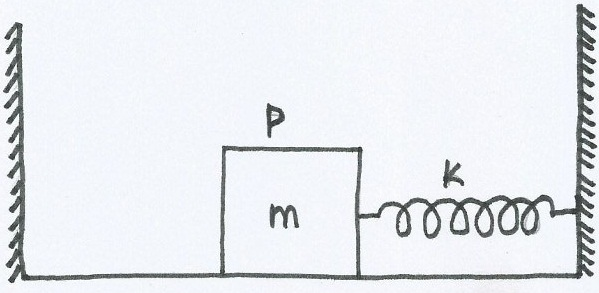# Spring-Block Systems!In the given figure a block P of mass $m$ is attached to a spring and is resting on a smooth horizontal floor at a distance $L$ from the left wall. Initially, the spring is in its natural length. P is now pushed towards right by a distance $\frac{3L}{2}$ and released. When block passes through the spring's relaxed state, another block of mass $m_1$ is placed on it which sticks to it. What is the value of $m_1$ so that the combined block just collides with the left wall?

×